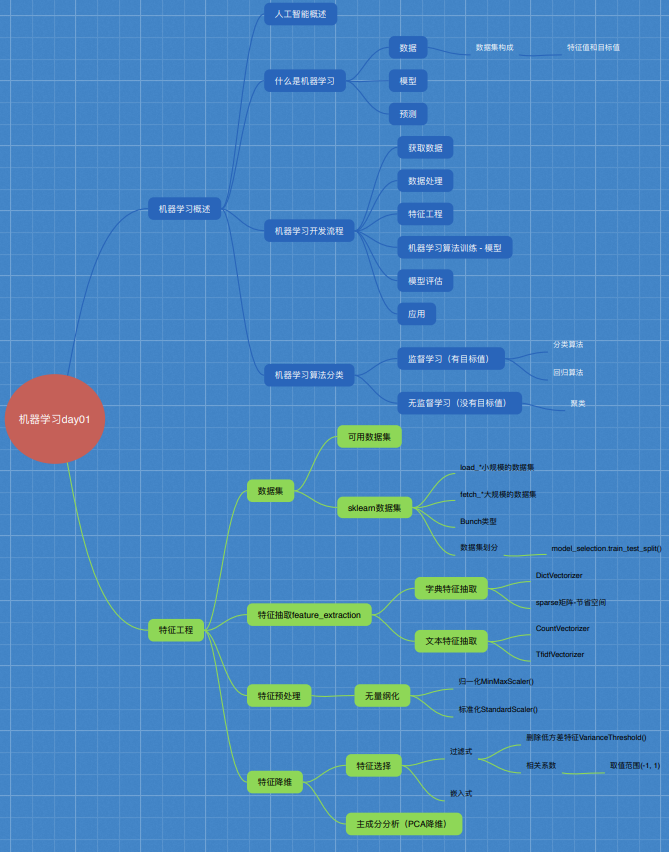# 一、机器学习概述

## 1.1 人工智能概述

### 1.1.1 机器学习与人工智能、深度学习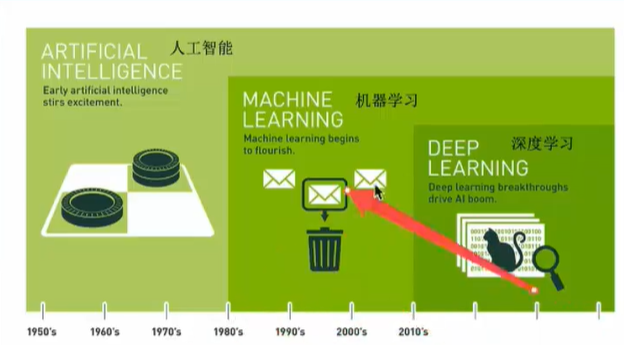• 机器学习是人工智能的一个实现途径
• 深度学习是机器学习的一种方法

### 1.1.2 机器学习、深度学习能做什么

• 传统预测：店铺销量预测、量化投资、广告推荐、企业客户分类、SQL语句安全检测分类
• 图像识别：街道交通标志检测、人脸识别
• 自然语言处理：文本分类、情感分析、自动聊天、文本检测

### 1.1.3 人工智能阶段课程安排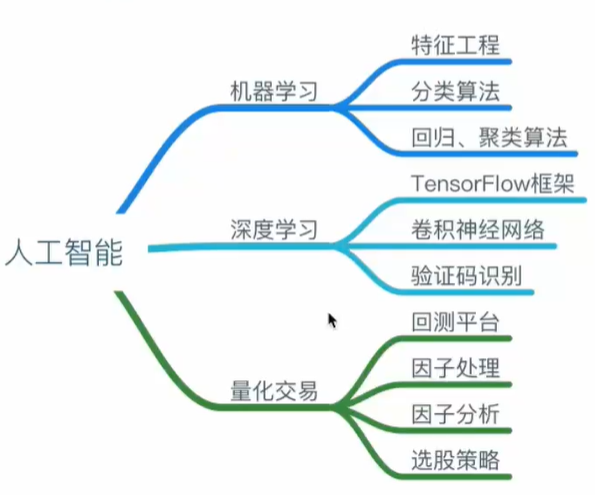## 1.2 什么是机器学习

### 1.2.2 解释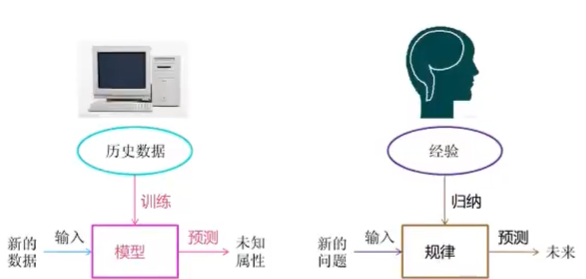### 1.2.3 数据集构成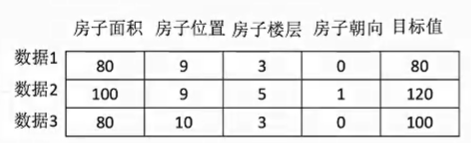• 对于每一行数据我们可以称为样本
• 有些数据集可以没有目标值## 1.3 机器学习算法分类### 1.3.1 总结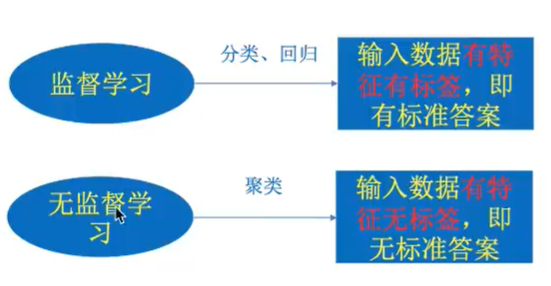### 1.3.2 练习

1、预测明天的天气是多少度？ 回归
2、依存明天是阴天还是晴天还是雨天？分类
3、人脸年龄预测？分类或回归
4、人脸识别？分类

### 1.3.3 机器学习算法分类

• 定义：输入数据是由输入特征和目标值所组成，函数的输出可以是一个连续的值，称为回归；也可以是一个离散的值，称为分类
• 分类：k-近邻算法、贝叶斯分类、决策树与随机森林、逻辑回归
• 回归：线性回归、岭回归

• 定义：输入数据是由输入特征值组成
• 聚类：k-means

## 1.4 机器学习开发流程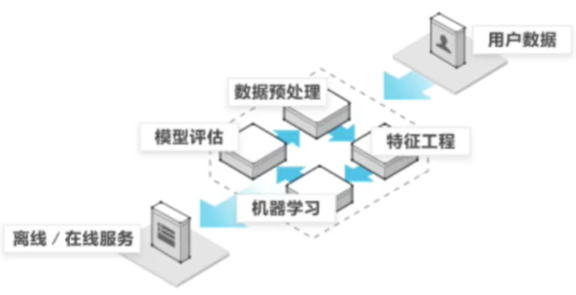1）获取数据
2）数据处理
3）特征工程
4）机器学习算法训练-得到模型
5）模型评估
6）应用

## 1.5 学习框架和资料介绍

• 分析很多的数据
• 分析具体的业务
• 应用常见的算法
• 特征工程、调参、优化

### 1.5.1 机器学习库与框架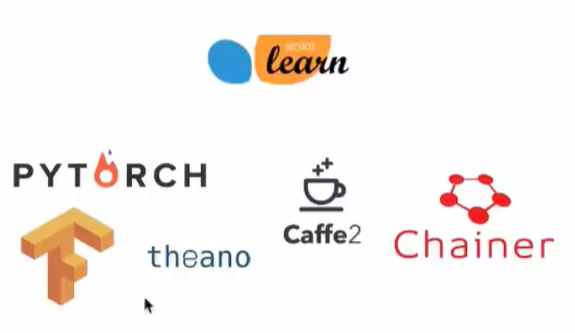### 1.5.2 书籍资料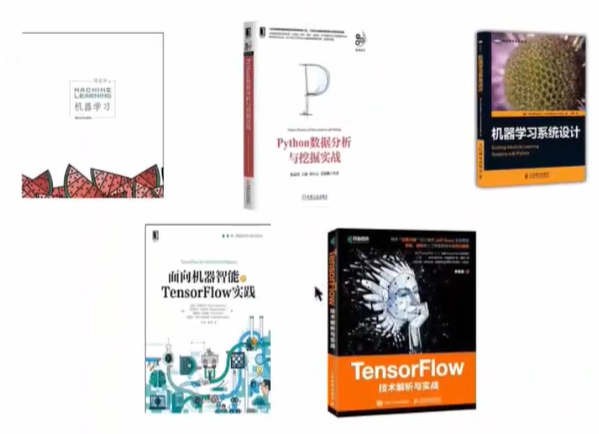### 1.5.3 提深内功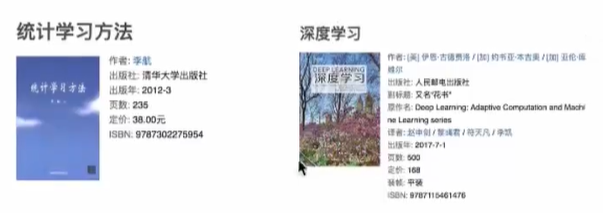# 二、特征工程

## 2.1 数据集

• 知道数据集分为训练集和测试集
• 会使用sklearn的数据集

### 2.1.1 可用数据集

Kaggle网址：https://www.kaggle.com/datasets
UCI数据集网址： http://archive.ics.uci.edu/ml/
scikit-learn网址： http://scikit-learn.org/stable/datasets/index.html#datasets
https://scikit-learn.org.cn/

scikit-learn特点：

• 1、数据量小
• 2、方便学习

UCI特点：

• 1、收录了360个数据集
• 2、覆盖科学、生活、经济等领域
• 3、数据集几十万

Kaggle特点：

• 1、大数据竞赛平台
• 2、80万科学家
• 3、数据量巨大

1 Scikit-learn工具介绍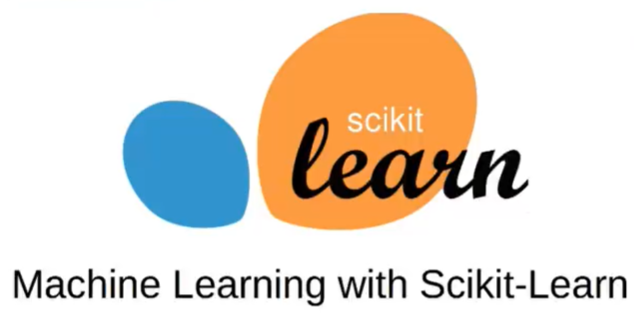• Python语言的机器学习工具
• Scikit-learn包括许多知名的机器学习算法的实现
• Scikit-learn文档完善，容易上手，丰富的API
• 目前稳定版本0.19.1

2 安装

``````pip install Scikit-learn -i https://pypi.douban.com/simple
``````

``````import sklearn
``````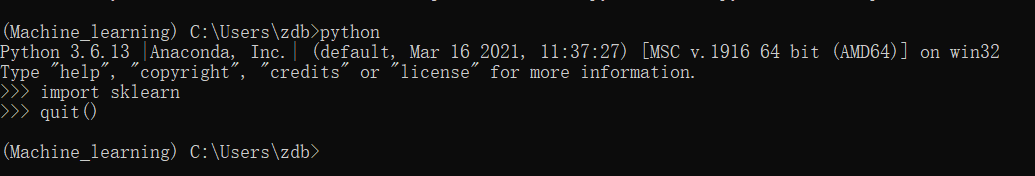3 Scikit-learn包含的内容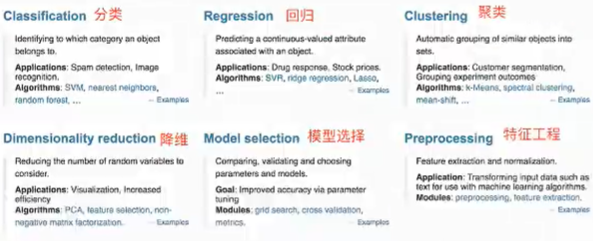### 2.1.2 sklearn数据集

1 scikit-learn数据集API介绍

• sklearn.datasets.fetch_*(data_home=None)：获取大规模数据集，需要从网络上下载，函数的第一个参数是data_home，表示数据集下载的目录，默认是**~/scikit_learn_data/**

2 sklearn小数据集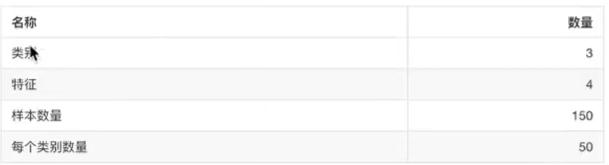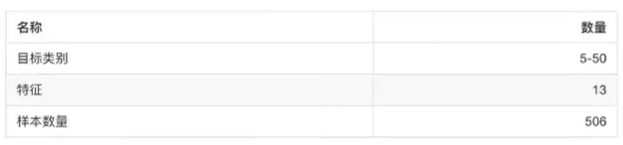3 sklearn大数据集
sklearn.datasets.fetch_20newsgroups(data=None, subset=‘train’)

• subset：‘train’或者’test’，‘all’，可选，选择要加载的数据集
• 训练集的‘训练’，测试集的‘测试’，两者的‘全部’

4 sklearn数据集的使用
sklearn数据集返回值介绍

• data：特征数据数组，是[n_samples * n_features]的二维numpy.ndarry数组
• target：标签数组，是n_samples的一维numpy.ndarry数组
• DESCR：数据描述
• feature_names：特征名，新闻数据，手写数字、回归数据集没有
• target_names：标签名
``````from sklearn.datasets import load_iris

def datasets_demo():
"""
sklearn数据集使用
:return:
"""
# 获取数据集
print("鸢尾花数据集：\n", iris)
print("查看数据集描述：\n", iris["DESCR"])           # 数据集的描述信息
print("查看特征值的名字：\n", iris.feature_names)
print("查看特征值：\n", iris.data, iris.data.shape)  # shape:(150,4)
return None

if __name__ == "__main__":
datasets_demo()
``````
``````查看特征值的名字：
['sepal length (cm)', 'sepal width (cm)', 'petal length (cm)', 'petal width (cm)']
``````

### 2.1.3 数据集的划分

• 训练数据：用于训练，构建模型
• 测试数据：在模型检验时使用，用于评估模型是否有效

• 训练集：70%、80%
• 测试集：30%、20%

• x 数据集的特征值
• y 数据集的标签值
• test_size测试集的大小，一般为float
• random_state随机数种子，不同的种子会造成不同的随机采样结果。相同的种子采样结果相同
• return训练集特征值，测试集特征值，训练集目标值，测试集目标值
``````from sklearn.datasets import load_iris
from sklearn.model_selection import train_test_split

def datasets_demo():
"""
sklearn数据集使用
:return:
"""
# 获取数据集
print("鸢尾花数据集：\n", iris)
print("查看数据集描述：\n", iris["DESCR"])
print("查看特征值的名字：\n", iris.feature_names)
print("查看特征值：\n", iris.data, iris.data.shape)  # 150个样本

# 数据集划分  X为特征 Y为标签
x_train, x_test, y_train, y_test = train_test_split(iris.data, iris.target, test_size=0.2, random_state=22)
print("训练集的特征值：\n", x_train, x_train.shape)   # 120个样本
return None

if __name__ == "__main__":
datasets_demo()
``````

## 2.2 特征工程介绍

### 2.2.3 特征工程的位置与数据处理的比较

• pandas：一个数据读取非常方便以及基本的处理格式的工具
• sklearn：对于特征的处理提供了强大的接口

• 特征抽取/特征提取
• 特征预处理
• 特征降维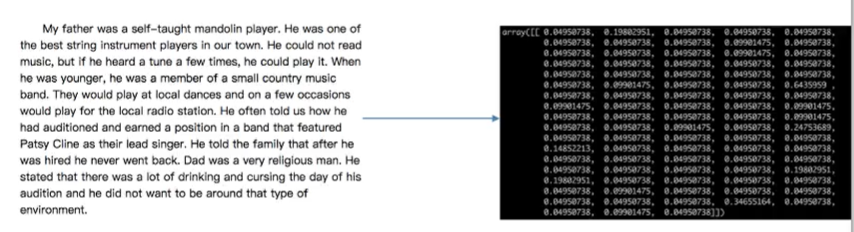## 2.3 特征提取

### 2.3.1 什么是特征提取

1 将任意数据（如文本或图像）转换为可用于机器学习的数字特征

• 字典特征提取（特征离散化）
• 文本特征提取
• 图像特征提取（深度学习再介绍）

2 特征提取API

``````sklearn.feature_extraction
``````

### 2.3.2 字典特征提取

sklearn.feature_extraction.DictVectorizer(sparse=True, …)

• DictVectorizer.fit_transform(X)， X：字典或者包含字典的迭代器返回值，返回sparse矩阵
• DictVectorizer.inverse_transform(X)， X：array数组或者sparse矩阵 返回值：转换之前数据格式
• DictVectorizer.get_feature_names()：返回类别名称

1 应用

``````from sklearn.feature_extraction import DictVectorizer

def dict_demo():
"""
字典特征抽取
:return:
"""
data = [{'city':'北京', 'temperature':100},
{'city':'上海', 'temperature':60},
{'city':'深圳', 'temperature':30}]
# 1、实例化一个转换器类
#transfer = DictVectorizer() # 返回sparse矩阵
transfer = DictVectorizer(sparse=False)
# 2、调用fit_transform()
data_new = transfer.fit_transform(data)
print("data_new：\n", data_new)   # 转化后的
print("特征名字：\n", transfer.get_feature_names())

return None

if __name__ == "__main__":
dict_demo()
``````
``````data_new：
[[  0.   1.   0. 100.]
[  1.   0.   0.  60.]
[  0.   0.   1.  30.]]
特征名字：
['city=上海', 'city=北京', 'city=深圳', 'temperature']
``````

### 2.3.3 文本特征提取

sklearn.feature_extraction.text.CountVectorizer(stop_words=[])：返回词频矩阵

• CountVectorizer.fit_transform(X)，X：文本或者包含文本字符串的可迭代对象，返回值：返回sparse矩阵
• CountVectorizer.inverse_transform(X)，X：array数组或者sparse矩阵，返回值：转换之前数据格
• CountVectorizer.get_feature_names()：返回值：单词列表

sklearn.feature_extraction.text.TfidVectorizer

1 应用

``````from sklearn.feature_extraction.text import CountVectorizer

def count_demo():
"""
文本特征抽取：CountVectorizer
:return:
"""
data = ['life is short,i like like python',
'life is too long,i dislike python']
# 1、实例化一个转换器类
transfer = CountVectorizer()
# 2、调用fit_transform
data_new = transfer.fit_transform(data)
print("data_new：\n", data_new.toarray())  # toarray转换为二维数组
print("特征名字：\n", transfer.get_feature_names())

return None

if __name__ == "__main__":
count_demo()
``````
``````data_new：
[[0 1 1 2 0 1 1 0]
[1 1 1 0 1 1 0 1]]

['dislike', 'is', 'life', 'like', 'long', 'python', 'short', 'too']
``````

``````from sklearn.feature_extraction.text import CountVectorizer

def count_demo():
"""
文本特征抽取：CountVectorizer
:return:
"""
data = ['life is short,i like like python',
'life is too long,i dislike python']
# 1、实例化一个转换器类
transfer = CountVectorizer(stop_words=['is', 'too'])
# 2、调用fit_transform
data_new = transfer.fit_transform(data)
print("data_new：\n", data_new.toarray())  # toarray转换为二维数组
print("特征名字：\n", transfer.get_feature_names())

return None

if __name__ == "__main__":
count_demo()
``````
``````data_new：
[[0 1 2 0 1 1]
[1 1 0 1 1 0]]

['dislike', 'life', 'like', 'long', 'python', 'short']
``````

``````from sklearn.feature_extraction.text import CountVectorizer

def count_demo():
"""
文本特征抽取：CountVectorizer
:return:
"""
data = ['我 爱 北京 天安门',
'天安门 上 太阳 升']
# 1、实例化一个转换器类
transfer = CountVectorizer()
# 2、调用fit_transform
data_new = transfer.fit_transform(data)
print("data_new：\n", data_new.toarray())  # toarray转换为二维数组
print("特征名字：\n", transfer.get_feature_names())

return None

if __name__ == "__main__":
count_demo()
``````
``````data_new：
[[1 1 0]
[0 1 1]]

['北京', '天安门', '太阳']
``````

``````from sklearn.feature_extraction.text import CountVectorizer
import jieba

def count_chinese_demo2():
"""
中文文本特征抽取，自动分词
:return:
"""
data = ['一种还是一种今天很残酷，明天更残酷，后天很美好，但绝对大部分是死在明天晚上，所以每个人不要放弃今天。',
'我们看到的从很远星系来的光是在几百万年之前发出的，这样当我们看到宇宙时，我们是在看它的过去。',
'如果只用一种方式了解某件事物，他就不会真正了解它。了解事物真正含义的秘密取决于如何将其与我们所了解的事物相联系。']
data_new = []
for sent in data:
data_new.append(cut_word(sent))
print(data_new)

# 1、实例化一个转换器类
transfer = CountVectorizer()
# 2、调用fit_transform
data_final = transfer.fit_transform(data_new)
print("data_final:\n", data_final.toarray())
print("特征名字：\n", transfer.get_feature_names())

return None

def cut_word(text):
"""
进行中文分词：“我爱北京天安门” -> "我 爱  北京 天安门"
:param text:
:return:
"""

return ' '.join(jieba.cut(text))

if __name__ == "__main__":
count_chinese_demo2()
#print(cut_word('我爱北京天安门'))
``````
``````['一种 还是 一种 今天 很 残酷 ， 明天 更 残酷 ， 后天 很 美好 ， 但 绝对 大部分 是 死 在 明天 晚上 ， 所以 每个 人 不要 放弃 今天 。', '我们 看到 的 从 很 远 星系 来 的 光是在 几百万年 之前 发出 的 ， 这样 当 我们 看到 宇宙 时 ， 我们 是 在 看 它 的 过去 。', '如果 只用 一种 方式 了解 某件事 物 ， 他 就 不会 真正 了解 它 。 了解 事物 真正 含义 的 秘密 取决于 如何 将 其 与 我们 所 了解 的 事物 相 联系 。']
data_final:
[[2 0 1 0 0 0 2 0 0 0 0 0 1 0 1 0 0 0 0 1 1 0 2 0 1 0 2 1 0 0 0 1 1 0 0 1
0]
[0 0 0 1 0 0 0 1 1 1 0 0 0 0 0 0 0 1 3 0 0 0 0 1 0 0 0 0 2 0 0 0 0 0 1 0
1]
[1 1 0 0 4 2 0 0 0 0 1 1 0 1 0 1 1 0 1 0 0 1 0 0 0 1 0 0 0 2 1 0 0 1 0 0
0]]

['一种', '不会', '不要', '之前', '了解', '事物', '今天', '光是在', '几百万年', '发出', '取决于', '只用', '后天', '含义', '大部分', '如何', '如果', '宇宙', '我们', '所以', '放弃', '方式', '明天', '星系', '晚上', '某件事', '残酷', '每个', '看到', '真正', '秘密', '绝对', '美好', '联系', '过去', '还是', '这样']
``````

5 Tf-idf文本特征提取

• Tf-idf的主要思想是：如果某个词或短语在一篇文章中出现的概率高，并且在其他文章中很少出现，则认为此词或者短语具有很好的类别区分能力，适合用来分来
• Tf-idf作用：用以评估一字词对于一个文件集或一个语料库中的其中一份文件的重要程度
这种方法是计算特征词的重要程度的
TF-IDF:衡量重要程度
TF：词频
IDF：逆向文档频率，可以由总文件数目 / 包含该词语之文件的数目，再将得到的商取以10为底的对数得到
``````from sklearn.feature_extraction.text import CountVectorizer, TfidfVectorizer
import jieba

def cut_word(text):
"""
进行中文分词：“我爱北京天安门” -> "我 爱  北京 天安门"
:param text:
:return:
"""

return ' '.join(jieba.cut(text))

def tfidf_demo():
"""
用TF-IDF的方法进行文本特征抽取
:return:
"""
data = ['一种还是一种今天很残酷，明天更残酷，后天很美好，但绝对大部分是死在明天晚上，所以每个人不要放弃今天。',
'我们看到的从很远星系来的光是在几百万年之前发出的，这样当我们看到宇宙时，我们是在看它的过去。',
'如果只用一种方式了解某件事物，他就不会真正了解它。了解事物真正含义的秘密取决于如何将其与我们所了解的事物相联系。']
data_new = []
for sent in data:
data_new.append(cut_word(sent))
print(data_new)

# 1、实例化一个转换器类
transfer = TfidfVectorizer()
# 2、调用fit_transform
data_final = transfer.fit_transform(data_new)
print("data_final:\n", data_final.toarray())
print("特征名字：\n", transfer.get_feature_names())
return None

if __name__ == "__main__":
tfidf_demo()
#print(cut_word('我爱北京天安门'))
``````
``````['一种 还是 一种 今天 很 残酷 ， 明天 更 残酷 ， 后天 很 美好 ， 但 绝对 大部分 是 死 在 明天 晚上 ， 所以 每个 人 不要 放弃 今天 。', '我们 看到 的 从 很 远 星系 来 的 光是在 几百万年 之前 发出 的 ， 这样 当 我们 看到 宇宙 时 ， 我们 是 在 看 它 的 过去 。', '如果 只用 一种 方式 了解 某件事 物 ， 他 就 不会 真正 了解 它 。 了解 事物 真正 含义 的 秘密 取决于 如何 将 其 与 我们 所 了解 的 事物 相 联系 。']
data_final:
[[0.30847454 0.         0.20280347 0.         0.         0.
0.40560694 0.         0.         0.         0.         0.
0.20280347 0.         0.20280347 0.         0.         0.
0.         0.20280347 0.20280347 0.         0.40560694 0.
0.20280347 0.         0.40560694 0.20280347 0.         0.
0.         0.20280347 0.20280347 0.         0.         0.20280347
0.        ]
[0.         0.         0.         0.2410822  0.         0.
0.         0.2410822  0.2410822  0.2410822  0.         0.
0.         0.         0.         0.         0.         0.2410822
0.55004769 0.         0.         0.         0.         0.2410822
0.         0.         0.         0.         0.48216441 0.
0.         0.         0.         0.         0.2410822  0.
0.2410822 ]
[0.12826533 0.16865349 0.         0.         0.67461397 0.33730698
0.         0.         0.         0.         0.16865349 0.16865349
0.         0.16865349 0.         0.16865349 0.16865349 0.
0.12826533 0.         0.         0.16865349 0.         0.
0.         0.16865349 0.         0.         0.         0.33730698
0.16865349 0.         0.         0.16865349 0.         0.
0.        ]]

['一种', '不会', '不要', '之前', '了解', '事物', '今天', '光是在', '几百万年', '发出', '取决于', '只用', '后天', '含义', '大部分', '如何', '如果', '宇宙', '我们', '所以', '放弃', '方式', '明天', '星系', '晚上', '某件事', '残酷', '每个', '看到', '真正', '秘密', '绝对', '美好', '联系', '过去', '还是', '这样']
``````

## 2.4 特征预处理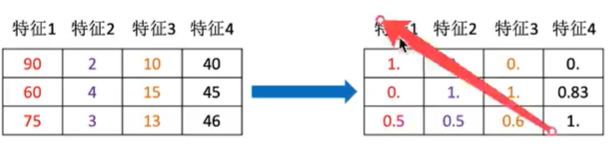### 2.4.1 什么是特征预处理

• 归一化
• 标准化

2 特征预处理API

``````sklearn.preprocessing
``````

• 特征的单位或者大小相差较大，或者某特征的方法相比其他的特征要大出几个数量级，容易影响（支配）目标结果，使得一些算法无法学习到其它的特征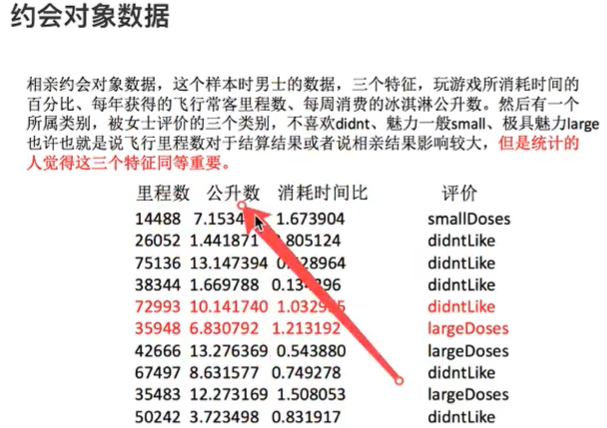### 2.4.2 归一化

1 定义

2 公式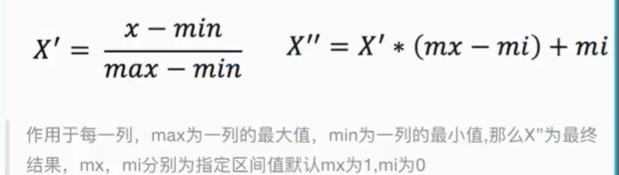3 API
sklearn.preprocessing.MinMaxScaler(feature_range=(0,1)…)
MinMaxScaler.fit_transform(X)，X：numpy array格式的数据[n_samples,n_features]，返回值：转换后的形式相同的array

4 数据计算

``````import pandas as pd
from sklearn.preprocessing import MinMaxScaler

def minmax_demo():
"""
归一化
:return:
"""
# 1、获取数据
data = data.iloc[:, :3]
print("data:\n", data)

# 2、实例化一个转换器类
transform = MinMaxScaler()
#transform = MinMaxScaler(feature_range=[2,3])

# 3、调用fit_transform
data_new = transform.fit_transform(data)
print("data_new:\n", data_new)

return None

if __name__ == "__main__":
minmax_demo()
``````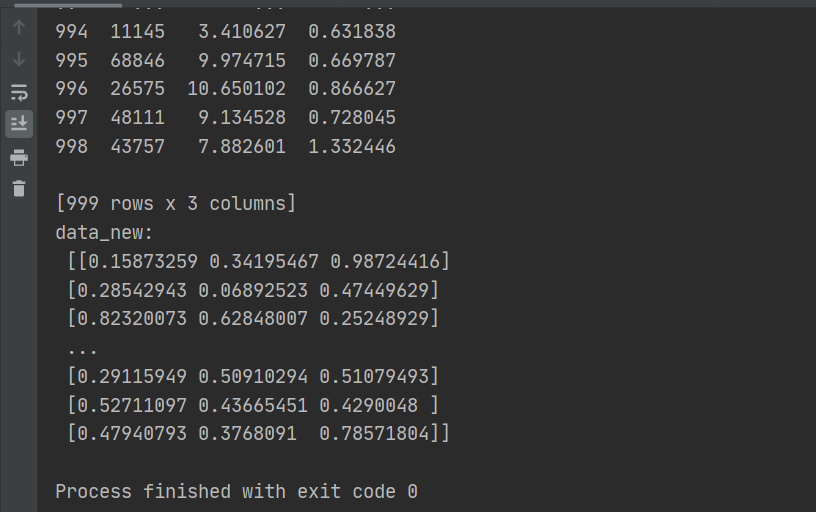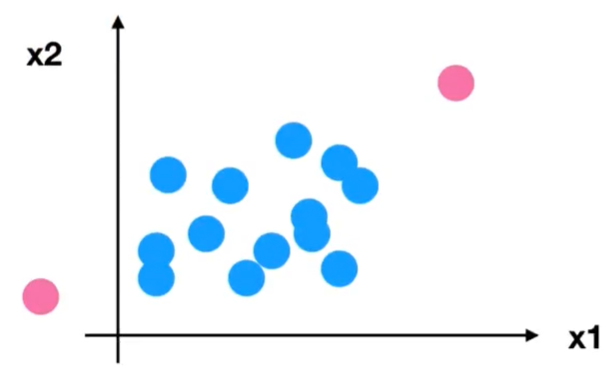5 归一化总结

### 2.4.3 标准化

1 定义

2 公式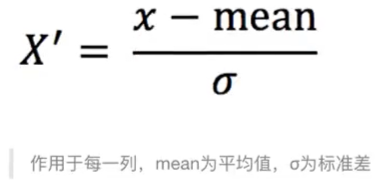• 对于归一化来说：如果出现异常点，影响了最大值和最小值，name结果显然会发生改变
• 对于标准化来说，如果出现异常点，由于具有一定数据量，少量的异常点对于平均值的影响不大，从而方差改变较小

4 代码

StandardScaler.fit_transform(X)，X;numpy array格式的数据[n_samples,n_features]，返回值：转化后的形状相同的array

``````from sklearn.preprocessing import MinMaxScaler, StandardScaler

def stand_demo():
"""
标准化
:return:
"""
# 1、获取数据
data = data.iloc[:, :3]
print("data:\n", data)

# 2、实例化一个转换器类
transform = StandardScaler()
#transform = StandardScaler(feature_range=[2,3])

# 3、调用fit_transform
data_new = transform.fit_transform(data)
print("data_new:\n", data_new)

return None

if __name__ == "__main__":
stand_demo()
``````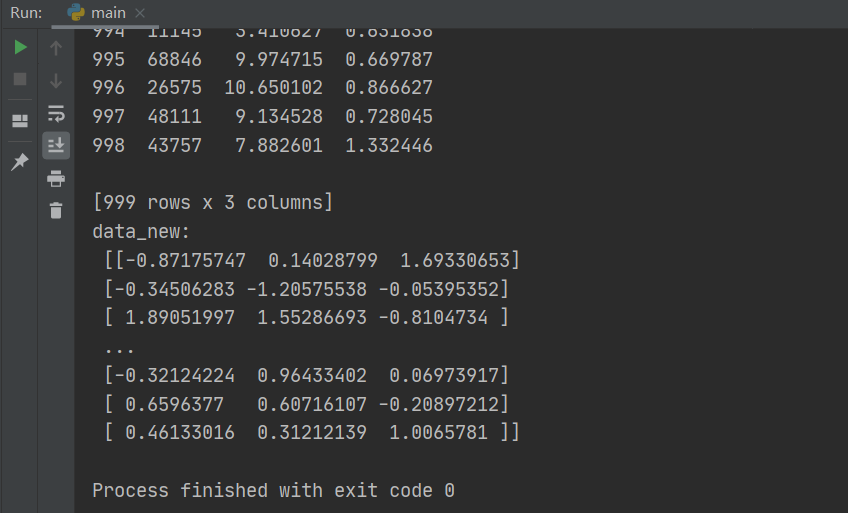5 标准化总结

## 2.5 特征降维

### 2.5.2 降维的两种方式

• 特征选择
• 主成分分析（可以理解一种特征提取的方式）

### 2.5.3 什么是特征选择

1 定义2 方法
Filter过滤式：主要探究特征本身特点、特征与特征和目标值之间关联

• （1）方差选择法：低方差特征过滤
• （2）相关系数：特征与特征之间的相关程度

Embedded嵌入式：算法自动选择特征（特征与目标值之间的关联）

• （1）决策树：信息熵、信息增益
• （2）正则化：L1，L2
• （3）深度学习：卷积等

3 模块

``````sklearn.feature_selection
``````

4 过滤式
4.1 低方差特征过滤

• 特征方差小：某个特征大多样本的值比较相近
• 特征方差大：某个特征很多样本的值都有差别

4.1.1 API
sklearn.feature_selection.VArianceThreshold(threshold=0.0)

4.1.2 数据计算

``````from sklearn.feature_selection import VarianceThreshold

def variance_demo():
"""
低方差特征过滤
:return:
"""
# 1、获取数据
print('data:\n', data)
data = data.iloc[:,1:-2]
print('data:\n', data)

# 2、实例化一个转换器类
#transform = VarianceThreshold()
transform = VarianceThreshold(threshold=10)

# 3、调用fit_transform
data_new = transform.fit_transform(data)
print("data_new\n", data_new, data_new.shape)

return None

if __name__ == "__main__":
variance_demo()
``````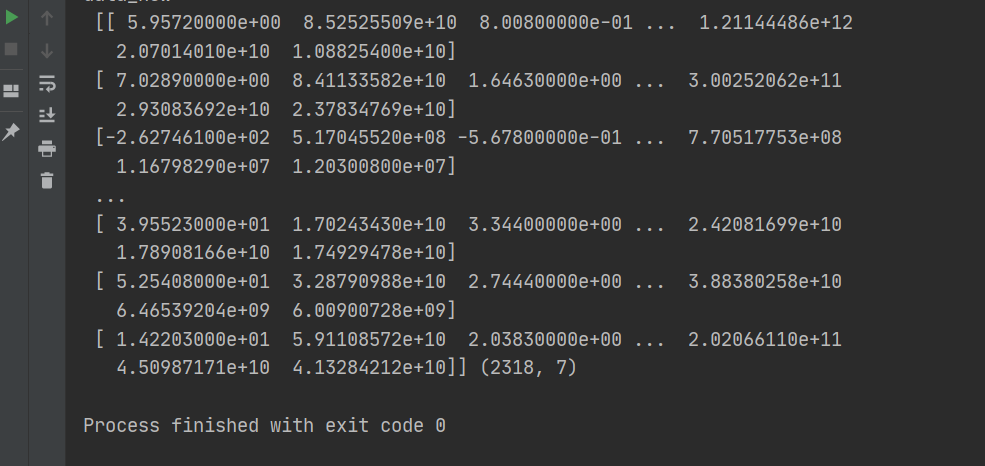4.2 相关系数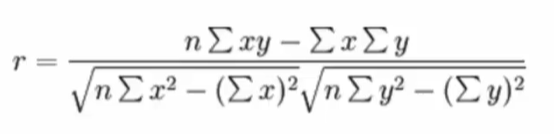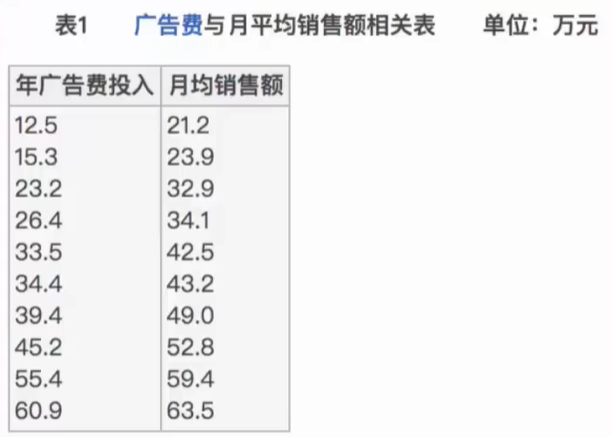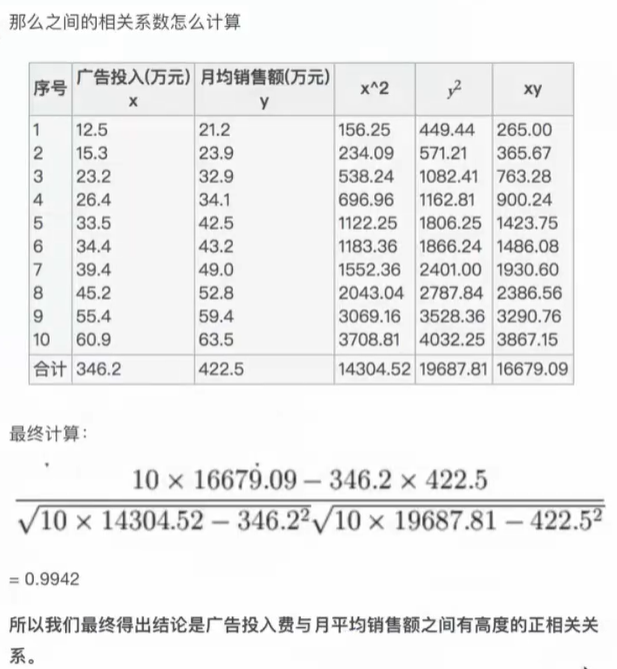4.2.3 特点

• 当r>0时，表示两变量正相关；r<0时，两变量为负相关
• 当|r|=1时，表示两变量为完全相关；当r=0时，表示两变量间无相关关系
• 当0<|r|<1时，表示两变量存在一定程度的相关。且|r|越接近1，两变量间线性关系越密切；|r|越接近0，表示两变量的线性相关越弱
• 一般可按三级划分：|r|<0.4为低度相关；0.4<=|r|<0.7为显著相关；0.7<=|r|<1为高维线性相关

4.2.4 API

`````` from scipy.stats import pearsonr
``````
• x：（N.）array_like
• y：（N.）array_like Returns:(Perason’s correlation coefficient, p-value)
``````from sklearn.feature_selection import VarianceThreshold
from scipy.stats import pearsonr

def variance_demo():
"""
低方差特征过滤
:return:
"""
# 1、获取数据
print('data:\n', data)
data = data.iloc[:,1:-2]
print('data:\n', data)

# 2、实例化一个转换器类
#transform = VarianceThreshold()
transform = VarianceThreshold(threshold=10)

# 3、调用fit_transform
data_new = transform.fit_transform(data)
print("data_new\n", data_new, data_new.shape)

# 计算两个变量之间的相关系数
r = pearsonr(data["pe_ratio"],data["pb_ratio"])
print("相关系数：\n", r)
return None

if __name__ == "__main__":
variance_demo()
``````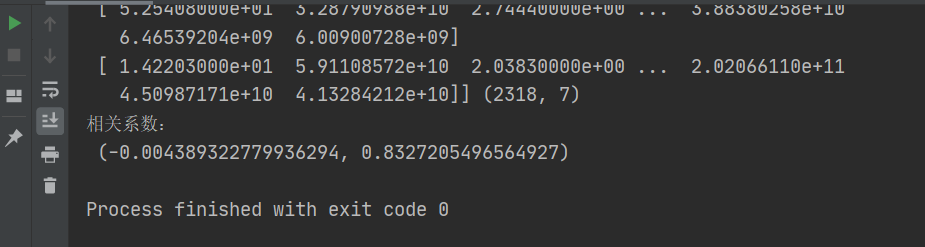1）选取其中一个
2）加权求和
3）主成分分析

## 2.6 主成分分析

### 2.6.1 什么是主成分分析（PCA）1 计算案例理解

2 代码
sklearn.decomposition.PCA(n_components=None)

• 将数据分解为较低维度空间
• n_components:
• 小数：表示保留百分之多少的信息
• 整数：减少到多少特征
PCA.fit_transform(X)，X：numpy array格式的数据[N_samples, n_features]，返回值：转换后指定维度的array

3 数据计算

``````from sklearn.decomposition import PCA

def pca_demo():
"""
PCA降维
:return:
"""
data = [[2,8,4,5], [6,3,0,8], [5,4,9,1]]
# 1、实例化一个转换器类
transform = PCA(n_components=2)  # 4个特征降到2个特征

# 2、调用fit_transform
data_new = transform.fit_transform(data)
print("data_new\n", data_new)

transform2 = PCA(n_components=0.95)  # 保留95%的信息
data_new2 = transform2.fit_transform(data)
print("data_new2\n", data_new2)

return None

if __name__ == "__main__":
pca_demo()
``````
``````data_new
[[ 1.28620952e-15  3.82970843e+00]
[ 5.74456265e+00 -1.91485422e+00]
[-5.74456265e+00 -1.91485422e+00]]
data_new2
[[ 1.28620952e-15  3.82970843e+00]
[ 5.74456265e+00 -1.91485422e+00]
[-5.74456265e+00 -1.91485422e+00]]
``````

### 2.6.2 案例：探究用户对物品类别的喜好细分降维

1）order_prodects_prior.csv：订单与商品信息

2）products.csv：商品信息
• 字段：product_id, product_name, aisle_id, department_id
3）order.csv：用户的订单信息
• 字段：order_id, user_id, eval_set, order_number, …
4）aisles.csv：商品所属具体物品类别
• 字段：aisle_id, aisle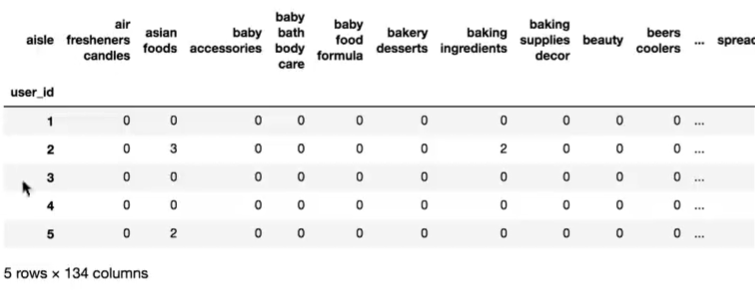1）需要将user_id和aisle放在同一个表中—合并
2）找到user_id和aisle----交叉表和透视表

``````# 1、获取数据
# 2、合并表
# 3、找到suer_id和aisle之间的关系
# 4、PAC降维
import pandas as pd
# 1、获取数据
orders = pd.read_csv('./instacart/orders.csv')     #3421083 rows × 7 columns
# 2、合并表'
​
# 合并aisles和products
tab1 = pd.merge(aisles, products, on=["aisle_id", "aisle_id"]) #49688 × 5 c
tab2 = pd.merge(tab1, order_products, on=["product_id", "product_id"])#32434489 ,8
tab3 = pd.merge(tab2, orders, on=["order_id", "order_id"])#32434489 ,14
# 3、找到suer_id和aisle之间的关系
table = pd.crosstab(tab3["user_id"], tab3["aisle"]) #206209 rows × 134 columns
data = table[:10000] #10000 rows × 134 columns

# 4、PAC降维
from sklearn.decomposition import PCA
# 1)实例化一个转换器类
transfer = PCA(n_components=0.95)  # 保留95%的信息
# 2）调用fit_transform
data_new = transfer.fit_transform(data)  #(10000, 42)，由134个特征降维到42个
``````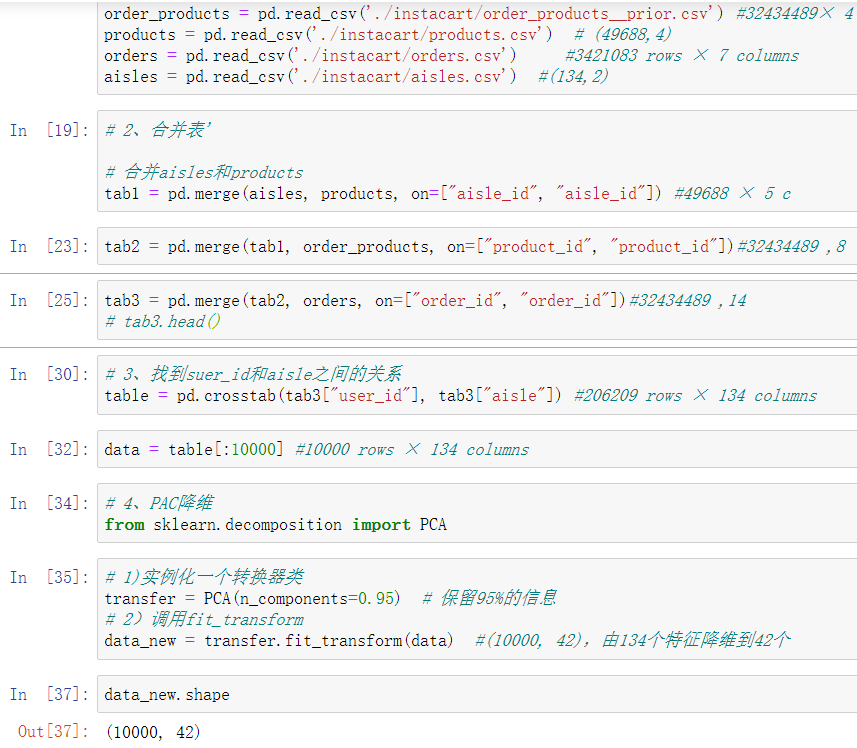## 2.7 机器学习第一天总结